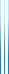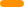# PublicationsPapers

## Bandwidth approximation of a restricted family of trees

Yuval Filmus
Master's thesis

We consider the NP-complete optimization problem Bandwidth. Anupam Gupta gave an $O(\log^{2.5} n)$ approximation algorithm for trees, and showed that his algorithm has an approximation ratio of $O(\log n)$ on caterpillars, trees composed of a central path and paths emanating from it. We show that the same approximation ratio is obtained on trees composed of a central path and caterpillars emanating from it.

Our result relies on the following lemma.

Definition. A sequence $a_1,\ldots,a_n$ has thickness $\Theta$ if the sum of any $d$ consecutive elements is at most $d\Theta$, for $1 \leq d \leq n$.

Lemma. If a sequence has thickness $\Theta$, then the sequence obtained by ordering the elements in non-decreasing order also has thickness $\Theta$.

## BibTeX

@mastersthesis{Filmus2002,
author = {Yuval Filmus},
title = {Bandwidth approximation of a restricted family of trees},
school = {Weizmann institute of science},
year = {2002}
}copy to clipboard